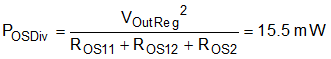SLUSD37E October   2017  – November 2019

PRODUCTION DATA.

1. Features
2. Applications
3. Description
1.      Device Images
4. Revision History
5. Device Comparison Tables
6. Pin Configuration and Functions
7. Specifications
8. Detailed Description
1. 8.1 Overview
2. 8.2 Functional Block Diagram
3. 8.3 Feature Description
1. 8.3.1 CrM/DCM Control Principle
2. 8.3.2 Line Voltage Feed-Forward
3. 8.3.3 Valley Switching and CrM/DCM Hysteresis
4. 8.3.4 Transconductance Amplifier with Transient Speed-up Function
5. 8.3.5 Faults and Protections
6. 8.3.6 High-Current Driver
4. 8.4 Controller Functional Modes
9. Application and Implementation
1. 9.1 Application Information
2. 9.2 Typical Application
1. 9.2.1 Design Requirements
2. 9.2.2 Detailed Design Procedure
1. 9.2.2.1 Custom Design With WEBENCH® Tools
2. 9.2.2.2 Power Stage Design
3. 9.2.2.3 ZCD/CS Pin
4. 9.2.2.4 VOSNS Pin
5. 9.2.2.5 Voltage Loop Compensation
3. 9.2.3 Application Curves
10. 10Power Supply Recommendations
11. 11Layout
1. 11.1 Layout Guidelines
2. 11.2 Layout Example
12. 12Device and Documentation Support
13. 13Mechanical, Packaging, and Orderable Information

• DBV|6

#### 9.2.2.4 VOSNS Pin

The VOSNS pin voltage is applied to the inverting input of an internal trasnconductance error amplifier. A fixed reference voltage (VOSReg) being applied to the non-inverting input. The error amplifier has high gain hence in steady-state, assuming VCOMP < 5-V, average voltage on the VOSNS pin must be approximately equal to the reference voltage (VOSReg). Output voltage regulation set point (VOutReg) is therefore determined by the external resistor divider network connecting the output voltage to the VOSNS pin according to the following expression.

Equation 70.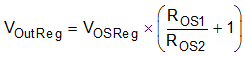The resistive divider that feeds the VOSNS pin makes a significant contribution to the unloaded input power. Higher resistor values reduce power consumption of the divider.

Equation 71.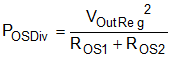Regulation accuracy degrades with increased resistor values due to the effect of VOSNS pin bias current (IOSBias).

Equation 72.To ensure that VOSNS pin bias current degrades output voltage regulation by less than 1%, the upper voltage divider resistor value must be constrained as show in Equation 73.

Equation 73.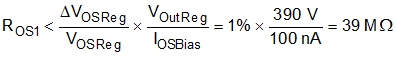Equation 73 confirms that reduction of the VOSNS divider dissipation to below 4 mW does not negatively affecting the regulation accuracy.

The PFC stage, of this design example, is to be followed by an LLC stage, that is controlled by UCC256301 device. The UCC28056 controller and the UCC256301 device operate together to form a complete off-Line power supply system with excellent light-load efficiency and standby power. To limit no-load input power a single resistor divider feeds both the VOSNS pin (UCC28056) and the BLK pin (UCC256301). A resistor divider with two taps is required because the UCC28056 requires a different divide ratio (KOS) to that required for the UCC256301 device (KBLK). The upper divider resistor (ROS1) is divided into two parts (ROS11, ROS12) to achieve the additional tap.

Equation 74.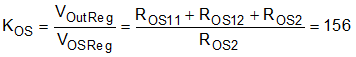Equation 75.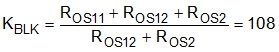For this design example select an upper divider resistor made up of three series-connected, 3.24-MΩ, 1206 SMT resistors. This compact and cost-effective design produces a suitable high-voltage resistor. If a single resistor is preferred, use a high voltage type, rated for the maximum voltage that can appear across the output capacitor during a Line surge test.

Equation 76.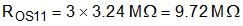Solving Equation 74 and Equation 75 simultaneously results in:

Equation 77.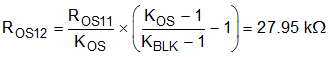Equation 78.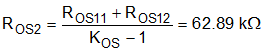These two divider resistor values can be implemented using easily obtainable values as follows:

Equation 79.Equation 80.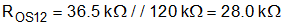Actual regulation set point is therefore:

Equation 81.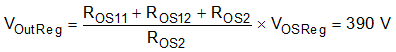Power dissipated in the VOSNS resistor divider is:

Equation 82.